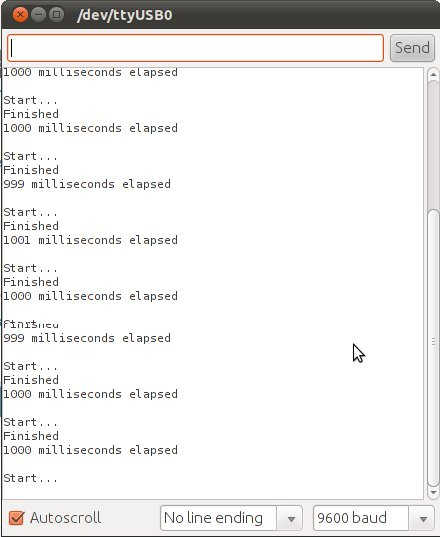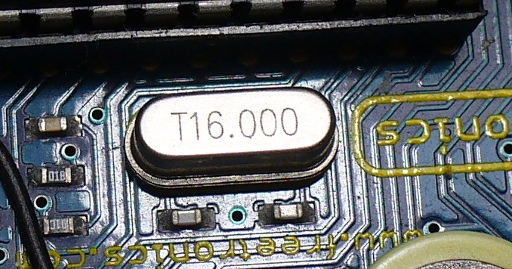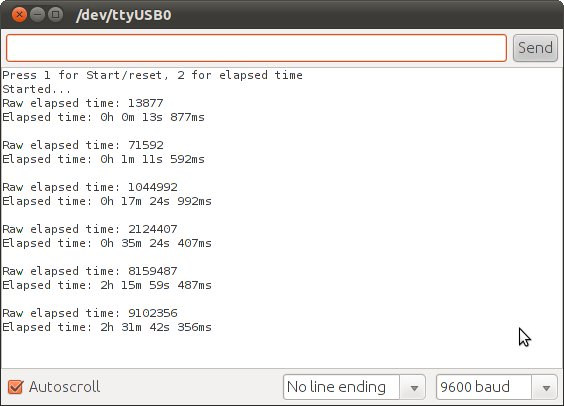Pi
2016.10.25 17:00

# [Arduino] millis() 함수로 시간 재기

조회 수 4292 댓글 0
?

#### 단축키

Prev이전 문서

Next다음 문서

+ - Up Down Comment Print
?

#### 단축키

Prev이전 문서

Next다음 문서

+ - Up Down Comment Print

http://tronixstuff.com/2011/06/22/tutorial-arduino-timing-methods-with-millis/

In this article we introduce the millis(); function and put it to use to create various timing examples.

Millis? Nothing to do with lip-syncers… hopefully you recognised milli as being the numerical prefix for one-thousandths; that is multiplying a unit of measure by 0.001 (or ten to the power of negative 3). Interestingly our Arduino systems will count the number of milliseconds (thousands of a second) from the start of a sketch running until the count reaches the maximum number capable of being stored in the variable type unsigned long (a 32-bit [four byte] integer – that ranges from zero to (2^32)-1.

(2^32)-1, or 4294967295 milliseconds converts to 49.71027-odd days. The counter resets when the Arduino is reset, it reaches the maximum value or a new sketch is uploaded. To get the value of the counter at a particular juncture, just call the function – for example:

 1 start=millis();

Where start is an unsigned long variable. Here is a very simple example to show you millis() in action:

 1234567891011121314151617181920212223242526 /*  Example 37.1 - millis() demonstration  http://tronixstuff.com/tutorials > chapter 37  John Boxall | CC by-sa-nc*/ unsigned long start, finished, elapsed; void setup(){  Serial.begin(9600);} void loop(){  Serial.println("Start...");  start=millis();  delay(1000);  finished=millis();  Serial.println("Finished");  elapsed=finished-start;  Serial.print(elapsed);  Serial.println(" milliseconds elapsed");  Serial.println();  delay(500);}

The sketch stores the current millis count in start, then waits one second, then stores the value ofmillis again in finished. Finally it calculates the elapsed time of the delay.  In the following screen dump of the serial monitor, you can see that the duration was not always exactly 1000 milliseconds:To put it simply, the millis function makes use of an internal counter within the ATmega microcontroller at the heart of your Arduino. This counter increments every clock cycle – which happens (in standardArduino and compatibles) at a clock speed of 16 Mhz. This speed is controlled by the crystal on the Arduino board (the silver thing with T16.000 stamped on it):Crystal accuracy can vary depending on external temperature, and the tolerance of the crystal itself. This in turn will affect the accuracy of your millis result. Anecdotal experience has reported the drift in timing accuracy can be around three or four seconds per twenty-four hour period. If you are using a board or your own version that is using a ceramic resonator instead of a crystal, note that they are not as accurate and will introduce the possibility of higher drift levels. If you need a much higher level of timing accuracy, consider specific timer ICs such as the Maxim DS3232.

Now we can make use of the millis  for various timing functions. As demonstrated in the previous example sketch, we can calculate elapsed time. To take this idea forward, let’s make a simple stopwatch. Doing so can be as simple or as complex as necessary, but for this case we will veer towards simple. On the hardware perspective, we will have two buttons – Start and Stop – with the 10k ohm pull-down resistors connected to digital pins 2 and 3 respectively.

When the user presses start the sketch will note the value for millis – then after stop is pressed, the sketch will again note the value for millis, calculate and display the elapsed time. The user can then press start to repeat the process, or stop for updated data. Here is the sketch:

 1234567891011121314151617181920212223242526272829303132333435363738394041424344454647484950515253545556 /*  Example 37.2 – Super-basic stopwatch using millis();  http://tronixstuff.com/tutorials > chapter 37  John Boxall | CC by-sa-nc*/ unsigned long start, finished, elapsed; void setup(){  Serial.begin(9600);  pinMode(2, INPUT); // start button  pinMode(3, INPUT); // stop button  Serial.println("Press 1 for Start/reset, 2 for elapsed time");} void displayResult(){  float h,m,s,ms;  unsigned long over;  elapsed=finished-start;  h=int(elapsed/3600000);  over=elapsed%3600000;  m=int(over/60000);  over=over%60000;  s=int(over/1000);  ms=over%1000;  Serial.print("Raw elapsed time: ");  Serial.println(elapsed);  Serial.print("Elapsed time: ");  Serial.print(h,0);  Serial.print("h ");  Serial.print(m,0);  Serial.print("m ");  Serial.print(s,0);  Serial.print("s ");  Serial.print(ms,0);  Serial.println("ms");  Serial.println();} void loop(){  if (digitalRead(2)==HIGH)  {    start=millis();    delay(200); // for debounce    Serial.println("Started...");  }  if (digitalRead(3)==HIGH)  {    finished=millis();    delay(200); // for debounce    displayResult();  }}

The calls to delay() are used to debounce the switches – these are optional and their use will depend on your hardware. Below is an example of the sketch’s serial monitor output – the stopwatch has started, and then button two pressed six times across periods of time:If you had a sensor at the start and end of a fixed distance, speed could be calculated: speed = distance ÷ time.

You can also make a speedometer for a wheeled form of motion, for example a bicycle. At the present time I do not have a bicycle to mess about with, however we can describe the process to do so – it is quite simple. (Disclaimer – do so at your own risk etc.)  First of all, let’s review the necessary maths. You will need to know the circumference of the wheel. Hardware – you will need a sensor. For example – a reed switch and magnet. Consider the reed switch to be a normally-open button, and connect as usual with a 10k ohm pull-down resistor. Others may use a hall-effect sensor – each to their own). Remember from maths class:(image licence)

To calculate the circumference – use the formula:

circumference = 2πr

where r is the radius of the circle. Now that you have the wheel circumference, this value can be considered as our ‘fixed distance’, and therefore the speed can be calculated by measuring the elapsed time between of a full rotation.

Your sensor – once fitted – should act in the same method as a normally-open button that is pushed every rotation. Our sketch will measure the time elapsed between every pulse from the sensor. To do this, our example will have the sensor output connected to digital pin 2 – as it will trigger an interrupt to calculate the speed. (Interrupts? See chapter three). The sketch will otherwise be displaying the speed on a normal I2C-interface LCD module. The I2C interface is suggested as this requires only 4 wires from the Arduino board to the LCD – the less wires the better.

Here is the sketch for your perusal:

 123456789101112131415161718192021222324252627282930313233343536373839404142434445464748495051 /*  Example 37.3 – Basic speedometer using millis();  http://tronixstuff.com/tutorials > chapter 37  John Boxall | CC by-sa-nc*/ #include "Wire.h" // for I2C bus LCD#include "LiquidCrystal_I2C.h" // for I2C bus LCD module - http://bit.ly/m7K5wtLiquidCrystal_I2C lcd(0x27,16,2);  // set the LCD address to 0x27 for a 16 chars and 2 line display float start, finished;float elapsed, time;float circMetric=1.2; // wheel circumference relative to sensor position (in meters)float circImperial; // using 1 kilometer = 0.621371192 milesfloat speedk, speedm;    // holds calculated speed vales in metric and imperial void setup(){  attachInterrupt(0, speedCalc, RISING); // interrupt called when sensors sends digital 2 high (every wheel rotation)  start=millis();  // setup LCD  lcd.init();      // initialize the lcd  lcd.backlight(); // turn on LCD backlight  lcd.clear();  lcd.println(" Wear a helmet! ");  delay(3000);  lcd.clear();  Serial.begin(115200);  circImperial=circMetric*.62137; // convert metric to imperial for MPH calculations} void speedCalc(){  elapsed=millis()-start;  start=millis();  speedk=(3600*circMetric)/elapsed; // km/h  speedm=(3600*circImperial)/elapsed; // Miles per hour} void loop(){  lcd.setCursor(0,0);  lcd.print(int(speedk));  lcd.print(" km/h ");  lcd.print(int(speedm));  lcd.print(" MPH   ");  lcd.setCursor(0,1);  lcd.print(int(elapsed));  lcd.print(" ms/rev      ");  delay(1000); // adjust for personal preference to minimise flicker}

There isn’t that much going on – every time the wheel completes one revolution the signal from the sensor will go from low to high – triggering an interrupt which calls the function speedCalc(). This takes a reading of millis() and then calculates the difference between the current reading and the previous reading – this value becomes the time to cover the distance (which is the circumference of the wheel relative to the sensor – stored in

 1 float circMetric=1.2;

and is measured in metres). It finally calculates the speed in km/h and MPH. Between interrupts the sketch displays the updated speed data on the LCD as well as the raw time value for each revolution for curiosity’s sake. In real life I don’t think anyone would mount an LCD on a bicycle, perhaps an LED display would be more relevant.

In the meanwhile, you can see how this example works in the following short video clip. Instead of a bike wheel and reed switch/magnet combination, I have connected the square-wave output from a function generator to the interrupt pin to simulate the pulses from the sensor, so you can get an idea of how it works:

That just about sums up the use of millis() for the time being. There is also the micros(); functionwhich counts microseconds. So there you have it – another practical function that can allow more problems to be solved via the world of Arduino. As always, now it is up to you and your imagination to find something to control or get up to other shenanigans.Have fun and keep checking into tronixstuff.com. Why not follow things on twitterGoogle+, subscribe  for email updates or RSS using the links on the right-hand column, or join our Google Group – dedicated to the projects and related items on this website. Sign up – it’s free, helpful to each other –  and we can all learn something.

# Dreamy의 코드 스크랩

## 내가 모으고 내가 보는

List of Articles
번호 분류 제목 날짜 조회 수 추천 수
387 Pi 시프트 레지스터 사용방법 (Shift Register, 74HC595, 74HC165) 2016.11.21 5902 0
386 Pi 커패시터 용량, 오차 및 정격전압 판별법 (Capacitor Code)2016.11.21 5699 0
385 Pi Simple DAC - PWM을 Voltage로 변환하는 회로2016.11.21 2638 0
384 Pi [Arduino] 아두이노 레오나르도 Arduino Leonardo Spec2016.11.17 5186 0
383 일반 18650 리튬이온 배터리 2016.11.15 7463 0
382 Pi 아두이노 (Arduino) 소개 및 유형별 종류, 제품별 특징 2016.11.15 2903 0
381 Pi 부품 구매 2016.11.15 2351 0
380 Android git 기초 - 원격 저장소 만들고 로그인하기, 소스 되돌리기 2016.10.29 5386 0
» Pi [Arduino] millis() 함수로 시간 재기 2016.10.25 4292 0
378 Pi [Arduino] Timer 라이브러리 사용하기 2016.10.25 4567 0
377 일반 나사 규격 2016.10.19 6827 0
376 Pi [Arduino] 아두이노 나노 Arduino Nano Spec 12016.09.07 10026 0
375 업무 마이크로 버블 Micro Bubble 이란 2016.08.29 3220 0
374 업무 전자저널 이용안내2016.08.29 0 0
373 업무 H&A본부 교육 중 IoT / 로봇 관련 내용2016.08.29 0 0
목록
Board Pagination ‹ Prev 1 2 3 4 5 6 7 8 9 10 ... 31 Next ›
/ 31

### 나눔글꼴 설치 안내

#### 이 PC에는 나눔글꼴이 설치되어 있지 않습니다.

이 사이트를 나눔글꼴로 보기 위해서는
나눔글꼴을 설치해야 합니다.

설치 취소

Designed by sketchbooks.co.kr / sketchbook5 board skin

Sketchbook5, 스케치북5

Sketchbook5, 스케치북5

Sketchbook5, 스케치북5

Sketchbook5, 스케치북5